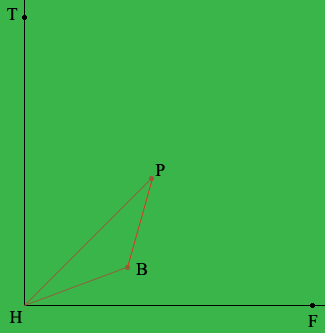SEARCH HOMEMath Central Quandaries & QueriesQuestion from ken, a student: A baseball player bunts a ball down the first base line. It rolls 35ft at an angle of 26 degrees with the first base path. The pitchers mound is 60.5 ft from the plate. How far must he travel to get to the ball?Hi Ken,

Draw a diagram!In my diagram (not to scale) F is first base, T is third base, H is home, P is the pitcher's mound and B is the ball. The measure of the angle BHF is 26 degrees. The measure of the angle PHF is 45 degrees. What is the measure of the angle PHB? The length of HB is 35 feet and the length of HP is 60.5 feet. You want to know the distance from P to B. Either the Law of Sines or the Law of Cosines should help here.

PennyMath Central is supported by the University of Regina and The Pacific Institute for the Mathematical Sciences.